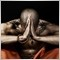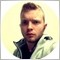# DLL string function does not work on Build 6001492

Hi coders!

I wrote a DLL function in Delphi. This code only return a PChar text to the ex4.

```ibrary get_text;

uses
SysUtils,
Math,
idHttp;

{\$R *.res}

var

function get_text(): PChar; stdcall;
begin
result := PChar('Hello world!');
end;

exports
get_text;

begin
end.```

My MQ4 code:

```#import "get_text.dll"
string get_text();
#import

int init(){

Print("DLL says: ",get_text());

}```

This solution works on Build 509, but on Build 600. On Build 600, the Experts output is: "DLL says: ???????????x??????????????"

Why does not work on B600? Have you got any idea to make my code works properly?

Relative276

Relative:

This solution works on Build 509, but on Build 600. On Build 600, the Experts output is: "DLL says: ???????????x??????????????"

v600 uses Unicode strings, not Ansi strings. You either need to return a Unicode string from your DLL (easier route), or you need to do some quite complex calls in the MQL4 code to manipulate the string return value (harder route).

Either way, this is going to leak memory because you are allocating a block of memory for the string which MT4 doesn't know how to free, and will not free. It is better to pass a buffer to the DLL, and have the DLL copy the string into that buffer. In other words, the better way of returning string values to MQL4 is to use the same sort of method as Windows API calls such as GetWindowsDirectory() and GetTempPath().276

gchrmt4:

or you need to do some quite complex calls in the MQL4 code to manipulate the string return value (harder route).

For the record, it is still possible to use DLLs which return Ansi strings; this is a sort of follow-up to https://www.mql5.com/en/forum/149321. The problem is that returning strings from DLLs leaks memory unless the MQL4 code knows how the memory was allocated and is able to free it. The following example assumes that the string memory was allocated in the DLL using LocalAlloc(), or some indirect equivalent which maps onto LocalAlloc().

```#import "SomeDllWhichReturnsAnsiStrings.dll"
// Declare the Ansi function as returning int rather than string
int Test();  // NOT  string Test();
#import

#import "kernel32.dll"
int lstrlenA(int);
void RtlMoveMemory(uchar & arr[], int, int);
int LocalFree(int); // May need to be changed depending on how the DLL allocates memory
#import

void OnStart()
{
// Call the DLL function and get its block of string memory as an int pointer to the
// memory rather than as a string
int ptrStringMemory = Test();

// Get the length of the string
int szString = lstrlenA(ptrStringMemory);

// Create a uchar[] array whose size is the string length (plus null terminator)
uchar ucValue[];
ArrayResize(ucValue, szString + 1);

// Use the Win32 API to copy the string from the block returned by the DLL
// into the uchar[] array
RtlMoveMemory(ucValue, ptrStringMemory, szString + 1);

// Convert the uchar[] array to a MQL string
string strValue = CharArrayToString(ucValue);

// Free the string memory returned by the DLL. This step can be removed but, without it,
// there will be a memory leak.
// The correct method for freeing the string *depends on how the DLL allocated the memory*
// The following assumes that the DLL has used LocalAlloc (or an indirect equivalent). If not,
// then the following line may not fix the leak, and may even cause a crash.
LocalFree(ptrStringMemory);

// Done...
Print(strValue);
}
```1492

Thank you gchrmt4!247

gchrmt4
:

For the record, it is still possible to use DLLs which return Ansi strings; this is a sort of follow-up to https://www.mql5.com/en/forum/149321. The problem is that returning strings from DLLs leaks memory unless the MQL4 code knows how the memory was allocated and is able to free it. The following example assumes that the string memory was allocated in the DLL using LocalAlloc(), or some indirect equivalent which maps onto LocalAlloc().

Thank you! It also worked for my libmysql.dll. My MQL4 can't talk to MySQL anymore since build 600

Finally I can at least see what MySql dll returns...

Could you give us a clue on how to implement the opposite communication, i.e. to pass a string from MQL4 (build 600) to dll that only supports ANSI? I tried UNICODE2ANSI() function found here and here but it doesn't work for me unfortunately.276

lukins:

Could you give us a clue on how to implement the opposite communication, i.e. to pass a string from MQL4 (build 600) to dll that only supports ANSI?

It's further down the same page! See https://www.mql5.com/en/forum/149321247

gchrmt4:
It's further down the same page! See https://www.mql5.com/en/forum/149321

Thank you! It works for simple string values just perfectly. At least MQL connects to MySQL now.

However seems like I can't get an array of strings from a dll. Here is the function I'm provided with (it's this MQL4 MySql wrapper):

```#import "mysql_wrapper.dll"
void     MT4_mysql_fetch_row   (int result, string& row[]);```

Currently I'm receiving "Access violation read to 0x0..." error when passing usual MQL's string array.

Any help is highly appreciated.247

Similar error (Access violation) occurs when I pass "int & row[]" and try to convert each element inside it after it's populated.276

lukins:

However seems like I can't get an array of strings from a dll. Here is the function I'm provided with (it's this MQL4 MySql wrapper):

Simulating old Ansi string arrays is messy, but still possible. (This is going to depend on the DLL being well-behaved, particularly if it passes data back to MQL4 by altering the contents of the array. I've only tested this against the example C++ code at the bottom, not against something more realistic like MySql library.)

```#import "SomeDllWhichTakesAStringArray.dll"
// Old Ansi function which takes a string array plus a parameter telling it
// the size of the array
void Test(int & arr[], int);  // NOT  void Test(string & arr[], int)
#import

#import "kernel32.dll"
int LocalAlloc(int,int);
int LocalFree(int);
int lstrcpyA(int,uchar & arr[]);
int lstrlenA(int);
void RtlMoveMemory(uchar & arr[], int, int);
#import

void OnStart()
{
// Example array of strings...
string MyStringArray[] = {"String 1", "String 2"};
int SizeOfStringArray = ArraySize(MyStringArray);

// A string array (received by the DLL as an array of MqlStr structures) corresponds
// to an int array where the even-numbered members are string lengths, and the odd-numbered
// members are pointers to string memory.
// We start by creating an int array, which needs to have twice as many members as the
// string array
int i;
int arrMqlStr[];
ArrayResize(arrMqlStr, SizeOfStringArray * 2);

// Populate the array which simulates MqlStr[]. For each string, we allocate
// a block of memory using LocalAlloc() and copy the MQL4 string into that
for (i = 0; i < SizeOfStringArray; i++) {
// Get the length of the string and store it
int szString = StringLen(MyStringArray[i]);
arrMqlStr[i * 2] = szString;

// Allocate a block of memory to hold the string
int ptrMem = LocalAlloc(0x40 /* LPTR */, szString + 1);
arrMqlStr[(i * 2) + 1] = ptrMem;

// Convert the MQL4 string to a uchar[] array
uchar ucString[];
StringToCharArray(MyStringArray[i], ucString);

// Copy the uchar[] array into the block of memory allocated above
lstrcpyA(ptrMem, ucString);
}

// Call the DLL function
Test(arrMqlStr, SizeOfStringArray);

// We now need to free the memory which was allocated above to hold
// a copy of each string. In addition, DLLs can alter strings which
// are passed to them in arrays. Therefore, for completeness, we
// should read the strings back and put them into the original
// array
for (i = 0; i < SizeOfStringArray; i++) {
// Get the size of the string now contained in the memory block
int NewSizeofString = lstrlenA(arrMqlStr[(i * 2) + 1]);

// Copy the contents of the memory block into a uchar[] array
RtlMoveMemory(ucReceive, arrMqlStr[(i * 2) + 1], NewSizeofString + 1);

// Put the uchar[] back into the original string array

// Free the memory for the string allocated above
LocalFree(arrMqlStr[(i * 2) + 1]);
}
}```

For example, the above code works with the following DLL which does a message box for each string in an array and then reverses the string before returning to MT4:

```__declspec(dllexport) void WINAPI Test(MqlStr * arr, int sz)
{
for (int i = 0; i < sz; i++) {
MessageBoxA(NULL, arr->data, "String", 48);
strrev(arr->data);
arr++;
}
}```276

gchrmt4:

Simulating old Ansi string arrays is messy, but still possible.

(The above code can be simplified if the MQL4 is passing the string array to the DLL only in order to give it a string buffer for the DLL to write into. The code above should handle that scenario, but it is unnecessarily complicated for a receive-only call to a DLL.)276

gchrmt4:
(The above code can be simplified if the MQL4 is passing the string array to the DLL only in order to give it a string buffer for the DLL to write into. The code above should handle that scenario, but it is unnecessarily complicated for a receive-only call to a DLL.)

If an Ansi DLL takes a string array parameter only so that it can pass back a value in arr, then the code can be much simpler. For example:

```#import "AnsiDllWhichPassesBackAStringValueViaAnArray.dll"
// Old Ansi function which takes a string array parameter **solely** so that
// it can **pass back** a value in arr
void Test(int & arr[]);  // NOT  void Test(string & arr[])
#import

#import "kernel32.dll"
int LocalAlloc(int,int);
int LocalFree(int);
void RtlFillMemory(int, int, int);
int lstrlenA(int);
void RtlMoveMemory(uchar & arr[], int, int);
#import

void OnStart()
{
// Maximum size of string which the DLL is expected to return in arr
int MaxReturnValueLength = 10000;

// Allocate a block of memory of the desired size
int ptrMem = LocalAlloc(0x40 /* LPTR */, MaxReturnValueLength + 1);

// Fill the memory with spaces so that the length is <whatever> if
// the DLL checks it by doing strlen()
RtlFillMemory(ptrMem, MaxReturnValueLength, 32);

// Create a pseudo-MqlStr array which corresponds to a string array with one member
int arrMqlStr;
arrMqlStr = MaxReturnValueLength;
arrMqlStr = ptrMem;

// Call the DLL
Test(arrMqlStr);

// Get the size of the string contained in the memory block
int NewSizeofString = lstrlenA(ptrMem);

// Copy the contents of the memory block into a uchar[] array

// Free the memory for the string allocated above
LocalFree(ptrMem);

// Convert the uchar[] array to a string
```__declspec(dllexport) void WINAPI Test(MqlStr * arr)